Geometric Calculator

Comments by: Odin Cary

This is one of the most overlooked commands in AutoCAD and unfortunately one of the most valuable tools.

Embedded within AutoCAD lies a calculator that cannot only be used for basic arithmetic functions, but also trigonometric functions, unit conversions and advanced geometric functions.

One function alone has made this tool invaluable to me and I will start with that simple routine.

1Example

Draw a square or rectangle.

Now put a circle in the exact center of that polygon.

Here's how you can do it with the Geometric Calculator: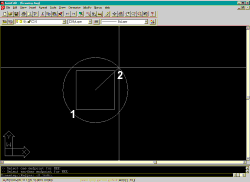Command: circle
CIRCLE 3P/2P/TTR/<Center point>: 'cal
Initializing...>> Expression: mee
>> Select one endpoint for MEE: (pick one corner)
>> Select another endpoint for MEE: (pick another corner diagonally opposite from the first)
Diameter/<Radius>:

What you have just done:

The Geometric Calculator was invoked transparently after the circle command ( 'cal ) and then utilized to perform a point calculation: the midpoint between two endpoints (hence the expression mee). You were queried for two points in exactly the same fashion you are queried for two object snap points. Notice that the Geometric Calculator quires for points with a pick box slightly larger than normal.

You can use this technique to find the midpoint between any two points anywhere in an AutoCAD drawing. It is fantastic when mirroring objects.

2Example

Have I gotten your attention yet?

Well, here's another great time saver.

Draw a horizontal line.

Draw another vertical line off of one of the endpoints of the previous horizontal line.

Now offset the last line 1/3 of the way down the horizontal line.

Here's how you can do it with the Geometric Calculator: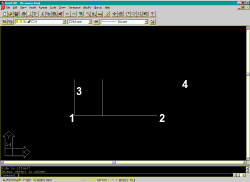Command:OFFSET
Offset distance or Through <Through>: 'cal
>> Expression: dist(end,end)/3
>> Select entity for END snap: ( pick one endpoint  )
>> Select entity for END snap:( pick the other endpoint )
Select object to offset: ( pick the vertical line  )
Side to offset? ( pick in the direction of offset  )
Select object to offset:

What you have just done:

The Geometric Calculator was invoked transparently after the Offset command and utilized to calculate the distance of the horizontal line, based upon its endpoints, and divided that distance by 3. The Offset command then received the calculated distance and allowed you to offset an object by that amount.

You can divide the distance by any number you want and you can also specify a distance by any object snap; e.g., dist(mid,quad)/10.

3General summary of the Geometric Calculator excerpted from class notes.

The Geometric Calculator:
A basic calculator and unit converter

Command: cal
>> Expression: cos(45)
0.707107

+, -, *, /, ^, (), pi

CVUNIT(value, unit_type, to what unit_type)

Command: cal
>> Expression: cvunit(2,m,ft)
6.56168

Acquiring the Midpoint of any two points in space.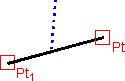Command: l
LINE From point: 'cal
>> Expression: (end+end)/2
>> Select entity for END snap:
>> Select entity for END snap:
To point:

Acquiring the Centroid of any REGULAR Polygon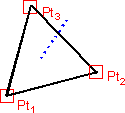Command:MOVE
Select objects: 1 found
Select objects:
Base point or displacement: 'cal
>> Expression: (end+end+end)/3
>> Select entity for END snap:
>> Select entity for END snap:
>> Select entity for END snap:
Second point of displacement:

Pre-designed Functions for the Geometric Calculator:

• MEE => Midpoint of two Endpoints => (end+end)/2
• ILLE => Intersection of two lines based upon selected endpoints
• ILL => same as ILLE but requires point specifications; e.g. ILL(int,end,mid,qua)
• DEE => Distance between two Endpoints => Dist(end,end)
• NEE => Normal vector off of two Endpoints => Nor(end,end)
• VEE => Vector off of two Endpoints => Vec(end,end)
• VEE1 => Unit Vector off of two Endpoints => Vec1(end,end)

These Functions can be used as per the following example: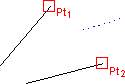Command: c
CIRCLE 3P/2P/TTR/<Center point>: 'cal
>> Expression: mee
>> Select one endpoint for MEE:
>> Select another endpoint for MEE:
Diameter/<Radius>:

Other Useful Functions for the Geometric Calculator

ANG(ApexPt1, Pt2, Pt3) => Returns the Angle derived by 3 points in space; e.g., ANG(int, end, end). Pt2 to Pt3 direction will determine value of angle relative to AutoCAD’s counter clockwise "Angle Direction" Default Setting.

PLD(Pt1, Pt2, Distance_Value) => Returns a point along a line based upon the Distance_Value relative to the First Point (Pt1) and the angle determined by the Second Point (Pt2 ); e.g., PLD(end,end,3) will return a point on a line 3 units from the First Point selected.